## 3A Microscopic Reaction-Diffusion Model for NBTI

As mentioned in Chap. 1, the reaction diffusion model has been mainly used for the interpretation of BTI experiments for almost four decades. Its apparent inability to explain NBTI recovery has led to several modifications of the concept, including trapping of the diffusing species and different types of diffusors .

 Figure 3.1: (left) According to Mahapatra et al.  , the inability of the macroscopic reaction-diffusion model (1.4)–(1.6) to predict the experimentally observed NBTI recovery is due to the one-dimensional description of the diffusive motion which makes it too easy for the hydrogen to find its dangling bond. (right) A correct description of the three-dimensional atomic motion, so the argument, leads to much richer repassivation kinetics and thus to a distribution of repassivation times.

Quite recently it was claimed that the misprediction of recovery is due to the one-dimensional description of the diffusing species in the macroscopic model (1.5)–(1.6). As illustrated in Fig. 3.1, it was suggested that this formulation makes it too easy for the hydrogen atom to find a dangling bond to passivate because the one-dimensional diffusion considers only two options of motion: forward and backward jumping. In a higher-dimensional description the diffusion and reaction kinetics are much richer:

1. The atoms can move in all three dimensions equally likely, leading to a distribution of arrival times at the interface during recovery.
2. H2-molecules dissociate at a dangling bond, creating a passivated dangling bond and a free hydrogen atom that does not immediately find another dangling bond to passivate.
3. Hydrogen atoms arriving later have to hover along the interface to find an unoccupied dangling bond.

 Figure 3.2: According to  , the distribution of repassivation times arising from an accurate three-dimensional microscopic description can be approximately modeled by varying the diffusion coefficient during recovery in the macroscopic model. The plot shows the numerically calculated recovery traces for different diffusion coefficients during recovery and the average of these traces. Indeed, this average shows a recovery that proceeds over more time-scales.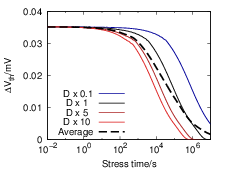A simple estimate of the recovery in the hypothetical three-dimensional model is given in  . This estimate mimics the different repassivation kinetics arising in the atomic description within the framework of the usual macroscopic RD model. To account for the longer “effective” recovery paths, the diffusion coefficients in the macroscopic model are reduced by different factors during recovery and the resulting recovery traces are averaged. Although this approach gives a recovery that proceeds over more time scales, as shown in Fig. 3.2, no derivation for the quasi-three-dimensional description is given and its physical validity is at least questionable. One of our targets is to test the claims of   within a firm theoretical framework.

 Figure 3.3: A random distribution of 10 dangling bonds on a silicon (100) surface corresponding to a dangling bond density of 5×1012cm-2, which is a common assumption for the number of bonding defects at the Si-SiO2 interface  [45, 20, 23]. The surface silicon atoms are shown in blue, the dangling bonds in red. At this density the average distance between two dangling bonds is ~ 4.5nm.

Another motivation for the study of the microscopic limit of the reaction-diffusion model for NBTI is based on general issues with the rate-equation-based description. As a literature study reveals, reaction-diffusion systems have been studied in numerous scientific communities from both the theoretical and the experimental side for more than a century . Although the mathematical framework of the RD model (1.4)–(1.6) seems physically sound and the description using densities and rate equations is commonly considered adequate, it is a well known and experimentally confirmed result of theoretical chemistry that the partial differential equation based description of chemical kinetics breaks down for low concentrations  . Additionally, in reaction-diffusion systems bimolecular reactions, such as the passivation and the dimerization reaction, require a certain proximity of the reactant species, termed reaction radius . Usually the elementary bimolecular reactions happen almost instantaneously and it is the required collision, i.e. the reduction of the distance between two reactants below the reaction radius, which is the rate limiting step . In chemical kinetics, these reactions are called diffusion-limited or diffusion-controlled reactions  .

It is easy to show that diffusion must play a dominant role in the bimolecular reactions in the RD model for NBTI. Fig. 3.3 schematically shows a uniform random distribution of dangling bonds on a silicon (100) surface that corresponds to a density of 5 × 1012cm-2, which is a usual assumption for N0   in (1.1) or (1.4). The average distance between two nearest neighbors at this density is d = N0-12 4.5nm. An atomic model of the Si-SiO2 interface as in Fig. 3.4 shows that two points separated by this distance have a large number of atoms in between. The assumption of an elementary reaction over this distance is clearly inappropriate, so any reaction between particles of this separation must involve a diffusive step.

Once established by the atomic viewpoint above, the diffusive influence on the bimolecular reactions leads to contradictions in the RD model and its physical interpretation. The predicted degradation of the RD model that is compatible with experimental data is only obtained if the hydrogen atoms that are liberated during stress compete for the available dangling bonds and dimerize at a certain rate. Both requirements involve diffusion over distances much larger than the nearest neighbor distance, which takes about two seconds at a commonly assumed diffusion coefficient of D = 10-13cm2s  . The reaction radius ρH of the dimerization reaction can be estimated from the Smoluchowski theory for irreversible bimolecular reactions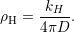(3.1)

While a reasonable reaction radius is in the regime of the average radius of the oxide interstitials, which is about 4Å , the application of (3.1) to published dimerization rates gives values ranging from 70μm for the parametrization of   to thousands of kilometers for other parametrizations  . Although both values for ρH seem quite unreasonable, they only indicate a limited physical validity of the selected parametrization. An evaluation of the physical validity of the reaction-diffusion model itself requires a more detailed study using a computational model that properly treats the stochastic chemical kinetics involved.

 Figure 3.4: An idealized atomic model of an MOS structure and the average dangling bond distance of 4.5nm, which spans several interstitial positions. It is intuitively clear that an elementary reaction between particles separated by this distance is strongly influenced by diffusion.For the present study of the microscopic properties of the RD mechanism we have developed our own atomistic reaction-diffusion simulator based on the considerations of the previous chapter. The microscopic reaction-diffusion picture developed for this purpose also serves as a framework to assess whether a description based on rate-equations is applicable to the physical mechanism behind the RD model.

3.2.2  Recovery

3.2.4  A Real-World Example

3.3  Related Work

### 3.1The Microscopic RD Model

Our microscopic RD model attempts to mimic the proposed mechanisms of the reaction-diffusion model at a microscopic level. The basic actors are H-atoms, H2-molecules and the silicon dangling bonds at the interface. The level of physical detail in our model is chosen so that the single-particle effects are captured in a physically meaningful way while still keeping the computational effort manageable even for long-term simulations. Atomic vibrations, i.e. the microstate dynamics, are certainly not of interest here. The present study concerns the RD-process itself and the conclusions therefore do not depend on the microscopic details of the reactions at its basis. The microscopic investigations are thus carried out at the stochastic chemistry level (see Chap. 2).

Several algorithms have been used in the chemical literature for the stochastic simulation of reaction-diffusion systems  . The most difficult task in the modeling of these systems is the diffusion of the reactants and several different approaches are available which can roughly be categorized as grid-based methods or grid-less methods  , see Fig. 3.5.

 Figure 3.5: Schematic illustration of the stochastic modeling approaches to reaction-diffusion systems. (left) In grid-less methods, a molecular trajectory is generated from the transient solution of Newton’s equations of motion as in molecular dynamics simulation. The interaction with the solvent is modeled by a random force that acts on the diffusors. Bimolecular reactions occur when two particles approach closer than the reaction radius (indicated as circles around the particles) (right) In grid-based methods diffusion proceeds as jumps between the sub-domains defined by the grid. Bimolecular reactions occur when two particles occupy the same grid-point.

Grid-less methods propagate the coordinates of the diffusing species quite similarly to molecular dynamics methods. Instead of explicitly treating all atoms of the solvent and their effect on the trajectory of the diffusors, the motion of the diffusing particles is perturbed by an empirical random force to generate a Brownian motion. Bimolecular reactions happen at a certain rate as soon as two reaction partners approach closer than a given radius. Although this technique suffers from its sensitivity to the time-step and the specific choice of the random force, it is a popular choice for the simulation of reaction-diffusion processes in liquid solutions where real molecular-dynamics simulations are not feasible . In grid-based methods the simulated volume is divided into small domains and each diffusing particle is assigned to a specific domain. The motion of the diffusors proceeds as hopping between the grid-points. In these models the bimolecular reactions happen at a certain rate as soon as two reactants occupy the same sub-volume. The advantage of this approach is that it can be formulated on top of the chemical master equation (2.20). The master equation can then be solved using the stochastic simulation algorithm (see Sec. 2.11), which does not depend on artificial time-stepping. A problem of the grid-based method that is repeatedly discussed in chemical literature is the choice of the spacial grid as it induces a more or less unphysical motion in liquid solutions. Additionally, the probability to find two particles in the same grid point and in consequence the rate of bimolecular reactions are quite sensitive to the volume of the sub-domains  .

In the reaction-diffusion model for NBTI, the diffusion of the particles proceeds inside a solid-state solvent. Contrary to diffusion in gases or liquids, the motion of an impurity in a solid-state host material proceeds via jumps between metastable states as illustrated in Fig. 3.6.

 Figure 3.6: Schematic microstate trajectory (blue) of an inert interstitial atom diffusing in a solid-state host material. The potential energy barriers of the host material are indicated as black grid. The diffusion itself proceeds via jumps between the interstitial positions. In between the jumps, the atom vibrates randomly around an energetic minimum.

This hopping diffusion is understood as a barrier-hopping process as explained in Sec. 2.8. In the case of H or H2, which do not react with the host atoms, the barriers arise from the repelling Coulomb-interaction between the electron clouds of the host lattice and the diffusor. The minima of the potential energy surface are thus the interstitial positions of the host lattice  . In between the jumps, the motion of the atom is randomly vibrational rather than diffusive or, in terms of Chap. 2, a microstate trajectory. This discreteness of motion not only strongly suggests the use of a grid-based method, where the grid points are interstitial positions of the host lattice, it also induces a natural discretization into the reaction-diffusion equations. As a consequence, the description based on macroscopic diffusion equations in the RD model (1.5)–(1.6) are only valid at distances that are much larger than the interstitial radius and it has to be assumed that at very short distances a description using hopping diffusion is more accurate.

We conclude that the most appropriate description of the physics considered in the present work is obtained from the reaction-diffusion master equation approach  . Within the natural lattice of interstitial positions the actors of our RD system exist in well-defined and discrete states. In accord with the considerations of Sec. 2.6, it is now possible to define a state vector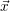that contains the interstitial positions and bonding states of all actors as well as a set of reaction channels which cause transitions between the states of this vector. The RD system then becomes a time-dependent stochastic process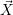(t) that exists in one of a countable set of states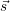ω and whose evolution over time can then be described by the chemical master equation (2.20). As explained in Sec. 2.6, the physics behind the reaction channels are contained in the propensity functions aγ and the state-change vectors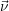γ, which are given in Fig. 3.7. The reactions employed in our simulations are the hopping transport between interstitial sites, the passivation/depassivation reaction and the dimerization/atomization reaction.

 Reaction Macroscopic Microscopic Illustration a. Si* + H→ SiH krNitHit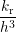nDBinHi SVG-Viewer needed. b. SiH →Si* + H kf(N0 - Nit) kfnp,i SVG-Viewer needed. c. H: I1 → I2 -D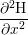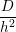nHi SVG-Viewer needed. d. H2 : I1 → I2 -D2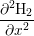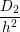nH2i SVG-Viewer needed. e. 2H → H2 kHH2 2nHi(nHi - 1) SVG-Viewer needed. f. H2 → 2H kH2H2 kH2nH2i SVG-Viewer needed.

…Dangling bond   …H   …H2

 Figure 3.7: Reaction channels and propensities in the microscopic RD model along with their macroscopic counterpart. (a) The dangling bonds are represented by special sites at the bottom of the simulation box. Empty dangling bond sites can be passivated by a free hydrogen atom. (b) Occupied dangling bond sites do not offer a bonding reaction channel, they can only emit their hydrogen atom. (c, d) Within the bulk SiO2, the atoms or molecules are allowed to jump from an interstitial I1 to any neighboring site I2. (e) When two hydrogen atoms occupy the same interstitial position, they can undergo a dimerization at rate kH and form H2. (f) Each hydrogen molecule dissociates at a rate kH2 back into two hydrogen atoms. For interstitial site i, nDBi is the number of (depassivated) dangling bonds, np,i is the number of passivating hydrogen atoms, nHi is the number of free hydrogen atoms, and nH2i is the number of hydrogen molecules. h denotes the step size of the spatial grid.

The stochastic chemical model is solved using the stochastic simulation algorithm (SSA) explained in Sec. 2.11.

 Figure 3.8: The simulation structure for the microscopic RD model employed in this work is a bounded region of width W and length L and infinite extension in z-direction, i.e. normal to the interface. The silicon dangling bonds are connected to special interstitial positions in the Si-SiO2 interface region at the bottom of the simulation box (blue). The interstitial positions are assumed to form an orthogonal lattice with constant jump-width and constant diffusion coefficients.

In the microscopic RD model employed in this work the interstitial sites form a regular and orthogonal three-dimensional grid and the hopping rates for the diffusors are assumed to be constant in accord with the isotropic and non-dispersive diffusion underlying the conventional macroscopic RD model . In a real SiO2 of a MOS transistor the amorphous structure will of course lead to a random network of interstitial sites  with a variety of hopping rates and a more complex topology. However, as the power-law degradation predicted by the macroscopic RD model requires a constant diffusion coefficient, these variations must be assumed unimportant  in order to obtain agreement with the established model. As illustrated in Fig. 3.8, the simulation region in our calculations is a rectangular box which extends to infinity normal to the Si-SiO2 interface and has closed lateral boundaries. The Si-SiO2 interface itself is represented by a special region at the bottom of the simulation box where selected interface sites have the ability to bond or release a diffusing hydrogen atom, see Fig. 3.7 and Fig. 3.8. The positions of the dangling bond sites in the interface region are picked randomly, similar to Fig. 3.3.

As mentioned above, the choice of the grid size requires special attention as it determines the probability of the bimolecular reactions. The interstitial size of amorphous silica has been calculated for molecular-dynamics generated atomic structures and is about 4Å . We take this value as the physically most reasonable grid size.

Once the microscopic model is defined, the relation to the macroscopic RD model (1.4)–(1.6) has to be established. Using the number of dangling bonds in the simulation box nDB, the number of hydrogen atoms passivating a dangling bond np and the numbers nHi of H and nH2i of H2 at interstitial i, this relation is obtained from the discretization induced by the grid  as

 N0 =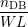, (3.2) Nit =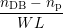, (3.3) H(xi) =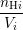, (3.4) H2(xi) =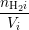, (3.5)
where W, L and h are illustrated in Fig. 3.8 and V i is the volume of interstitial i which is V i = h3 in this work. The relation between the rates of the macroscopic model and the microscopic propensity functions are given in Fig. 3.7. Initially, all hydrogen atoms are passivating silicon dangling bonds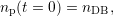(3.6)

in accordance with the assumptions of the macroscopic RD model.

### 3.2Results and Discussion

Two different systems have been studied in detail: a model system and a “real-world-example”. The model system is used to study the general features of the microscopic reaction-diffusion process. It is parametrized in order to clearly show all relevant features at a moderate computational effort. The parametrization of the real-world system is based on a published parametrization of the modified reaction-diffusion model. This system is used to relate our microscopic model to published data.

#### 3.2.1General Behavior of the Microscopic RD Model

 Table 3.1: Parameters of the model system. The parameters have been selected to enable a study of the different regimes of the microscopic RD model at moderate computational expense. The rates are given in terms of the microscopic model as in Fig. 3.7.
 Reaction Propensity Depassivation 0.5s-1 Passivation 4 × 104s-1 Dimerization 2 × 105s-1 Atomization 5s-1 H-hopping 100s-1 H2-hopping 100s-1

The parametrization that is used to study the general behavior is given in Tab. 3.1. The number of diffusing particles is a trade-off between accuracy and computational speed. Due to the large computational demand1 , different regimes of the degradation curve have to be calculated with different numbers of diffusing particles.

The earliest degradation times are dominated by the depassivation of the silicon dangling bonds leading to a linear increase of the degradation, which is equivalent to the initial “reaction limited” degradation of the macroscopic RD model . However, the degradation predicted by the microscopic RD model quickly saturates as an equilibrium forms between depassivation and repassivation for each dangling bond separately. In absence of any diffusion the time evolution of the number of hydrogen atoms passivating a silicon dangling bond is given by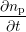(t) = -kfnp(t) +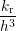(nDB - np(t)) (3.7) np(t = 0) = nDB. (3.8)
with the solution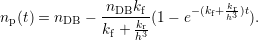(3.9)

The main difficulty in the calculation of the early degradation times in the microscopic RD model is the very low degradation level in this regime, which requires a high accuracy, i.e. a large number of particles to obtain smooth results. Fortunately, as reactions between the hydrogen atoms or between hydrogen atoms and neighboring dangling bonds are not happening in this regime, a good parallelization can be obtained by averaging over separate simulation runs, see Fig. 3.9.

 Figure 3.9: As the interaction between the diffusing particles is small at early degradation times, the computation can be parallelized by averaging over several simulation runs. The figure shows that a calculation with 105 particles is equivalent to the average of 100 calculation runs with 103 particles. The result of a single 103 particle run is shown for comparison. kf was increased by a factor of 100 for this calculation, in order to obtain smooth curves from the 105 particle run.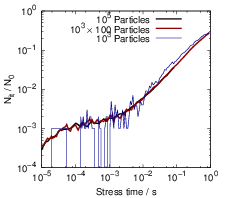A comparison of the microscopic RD model and (3.9) is shown in Fig. 3.10.

 Figure 3.10: Comparison of the microscopic RD model with 25000 particles averaged over 50000 runs to its macroscopic counterpart, the single-particle expressions (3.10)–(3.11) and the isolated dangling-bond equilibration (3.9). The earliest degradation times are dominated by the equilibration between the depassivation and passivation reaction at every dangling bond. Around 1ms, the departure of the hydrogen atoms from the dangling bond site begins but the interaction between the diffusors is still negligible.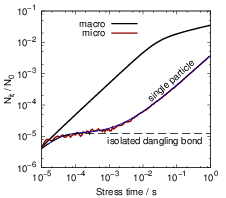The initial behavior of the microscopic RD model stands in stark contrast to the degradation in the macroscopic model where the linear regime continues until a global equilibrium has formed at the interface.

As this initial behavior takes a central position in our further discussion, it requires a deeper analysis. According to Sec. 2.12 the microscopic single-particle regime can be accurately described using rate equations, as it does not contain any second-order reactions. The required equations are basically those of the RD model, but as every hydrogen atom can be assumed to act independently, the expressions for the hydrogen bonding as well as the competition for dangling bonds are neglected. As the kinetic behavior in this regime is strongly determined by the first diffusive steps of the hydrogen atoms, the diffusion part of this approximation must have the same interstitial topology as the microscopic model. As all hydrogen atoms act independently, only one atom and one dangling bond need to be considered. The interface reaction and the diffusion of the hydrogen atom is thus described as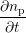= -kfnp +nDBnH0  and (3.10)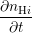= ∑ j∈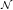(i)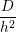(nHj - nHi), (3.11)
respectively, where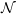denotes the set of neighboring interstitials to i. Fig. 3.10 compares the microscopic RD calculation with the approximations for the different regimes at early degradation times, which shows that the single-particle model perfectly matches the behavior of the atomic model in the initial phase.

After the atoms have traveled sufficiently long distances, the interaction between the particles becomes relevant and the single-particle approximation becomes invalid. In Fig. 3.11 this is visible as a transition away from the single-particle behavior towards the macroscopic solution between 1s and 1ks. Due to the relatively large level of degradation, the long-term simulations do not require as much accuracy as the short-term simulations. Consequently the number of particles can be reduced for longer simulation times, which makes the prediction of long-term degradation possible.

 Figure 3.11: Long-term simulations can be executed with lower accuracy due to the increased level of degradation. Three microscopic calculations are compared to the macroscopic result. The 25000 particle simulation nicely shows the transition between the single-particle and the macroscopic diffusion-limited regime. The 1000 particle calculation captures the transition region but becomes quite noisy for earlier degradation times. The 90 particle run still captures the macroscopic regime with reasonable accuracy.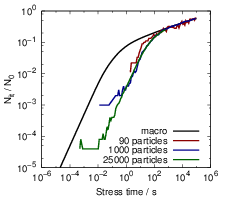Finally, Fig. 3.12 compares the microscopic RD model to the macroscopic version over the course of one complete stress cycle, where the microscopic curve was obtained by combining calculations of different accuracy, as explained above. Instead of the three regions which arise from the macroscopic RD model — reaction-limited, equilibration and diffusion-limited — the H-H2 microscopic description has four to five regimes depending on the particular parametrization.

• The earliest degradation times (t < 20μs in this case) are dominated by the depassivation of dangling bonds. In this regime, the microscopic and the macroscopic model give identical degradation behavior.
• After the passivation and depassivation have reached an equilibrium between kf and kr separately for each Si-H bond, the fraction of depassivated dangling bonds remains constant until the diffusion of the hydrogen atoms becomes dominant. This regime only shows when the individual hydrogen atoms are considered and consequently is not obtained from any model based on rate equations.
• As more and more hydrogen atoms leave their initial position, the degradation is determined by the buildup of a diffusion front along the Si-SiO2 interface and the equilibration between the dangling bonds. This regime has a very large power-law exponent of almost one2 that is not experimentally observed. The stress time range in which this regime is observed depends on the average distance between two dangling bonds, the diffusion coefficient and the interstitial size.
• As the bimolecular reactions become relevant, the macroscopic diffusion-limited regime begins to emerge. For some parametrizations we have observed a time window in which a sufficient amount of H2 has not formed yet, and thus the initial diffusion-limited regime has the typical t14-form that arises from the classical RD model without H2  .

The initial single-particle phase of the degradation is a remarkable feature of the microscopic model. As it is incompatible with experimental data and very sensitive to the parametrization, its relevance for real-world reliability projections has to be investigated. For this purpose we have run calculations based on a published parametrization of the reaction-diffusion model for NBTI, see Sec. 3.2.4.

 Figure 3.12: Comparison of all regimes of the microscopic RD model to the degradation predicted by the macroscopic RD model. Obviously there is a large discrepancy between the two descriptions and the behavior of the physically more reasonable microscopic model is not experimentally observed.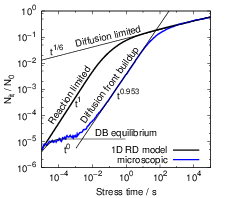#### 3.2.2Recovery

 Figure 3.13: Recovery transients for different stress times. As the degradation transient approaches the macroscopic diffusion limited regime (see inset), the recovery comes closer to the macroscopic recovery, leading to a perfect match as soon as the degradation assumes the experimentally relevant t1∕6 form.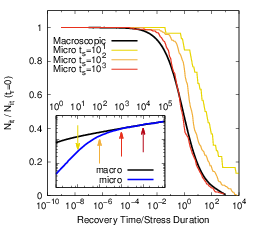In agreement with our investigations on two-dimensional systems  , the three-dimensional stochastic motion of the hydrogen atoms does not influence the recovery behavior of the system after long-term stress, which contradicts the suggestions of  . As shown in Fig. 3.13, a longer relaxation transient is only obtained if the foregoing stress phase does not show a power-law regime. As the system comes closer to the macroscopic degradation behavior, the recovery in the microscopic model also approaches the macroscopic version, which is incompatible with experimental data . This behavior is to be expected as the t16 degradation regime requires an equilibration and thus a quasi-one-dimensional behavior. The recovery proceeds on a timescale that is at least two orders of magnitude longer than the stress time. The lateral search of hydrogen atoms for unoccupied dangling bonds was suggested to dominate at the end of the recovery. However, due to the logarithmic time scale on which recovery is monitored, the equilibration along the interface has negligible impact at the end of the recovery trace if this equilibration proceeds about two orders of magnitude faster. Thus, the hovering of hydrogen atoms along the interface does not influence the shape of the recovery transient.

#### 3.2.3Approximations in the Macroscopic Model

After the microscopic RD theory Fig. 3.7 has been established and its general behavior has been investigated, one can use this framework to analyze the assumptions that are implicit to the macroscopic RD model (1.4)–(1.6), which is still widely considered to be an adequate approximation.

The most obvious approximation in the macroscopic RD model is the one-dimensional description of diffusion. While this may seem to be appropriate as boundary effects in the diffusion of both H and H2 are negligible, it tacitly introduces the assumption of lateral homogeneity along the interface. This homogeneity includes the following assumptions:

• All the liberated hydrogen atoms at the interface (Hit in (1.1) and (1.4)) compete instantaneously with all the other free interfacial hydrogen atoms for all the available dangling bonds.
• All the pairs of hydrogen atoms at a certain distance from the Si-SiO2 interface are equally likely to undergo dimerization and form H2, independently of their spatial separation.

As was shown above, a hydrogen atom liberated during stress initially stays in the vicinity of its original dangling bond and thus the lateral homogeneity has to be considered a long-term approximation. It is accurate when the diffusion of hydrogen has led to enough intermixing so that there is no significant variability in the concentration of free hydrogen along the interface. Following   and the discussion in Sec. 2.12, this condition can be called “lateral well-stirredness” of the system.

The second and more delicate approximation in the macroscopic RD model is the mathematical description using rate- and diffusion-equations. In the microscopic RD model, the rate at which an atom at the interface passivates a dangling bond depends not only on the rate kr but also on the probability of finding this atom at the position of the dangling bond. In the macroscopic model the precondition of having an unoccupied dangling bond at the interface is described multiplicatively as krNitHit. At early times during degradation, when each hydrogen atom still resides near its dangling bond, this term introduces an unphysical self-interaction where each hydrogen atom competes with itself for its dangling bond. As the root of this problem lies in the assumptions implicit to a formulation based on rate-equations, the error is also present in a macroscopic model with three-dimensional diffusion. As explained in  , this means that a rate-equation based RD model will not accurately describe the degradation at early times even if higher-dimensional diffusion and discrete dangling bonds are considered.

Similar to the passivation rate, the rate at which H2 is formed in the microscopic RD model depends on both the dimerization rate kH and the probability of finding two hydrogen atoms which occupy the same interstitial position. In the macroscopic RD model, this dimerization reaction is modeled as kHH2. As thoroughly explained in  , this approximation is only valid for large numbers of particles, as the number of pairs of hydrogen atoms in an interstitial goes as N(N - 1) which can only be approximated as N2 if N is sufficiently large.

All in all, the macroscopic RD model can only be considered a valid approximation of the microscopic RD model for very long stress times and a sufficient amount of liberated hydrogen atoms. The time it takes for the macroscopic approximation to become valid, however, may exceed the time range in which it is usually applied, depending on the parametrization.

#### 3.2.4A Real-World Example

 Table 3.2: The parameters employed in the real-world simulations. The parameter set is based on the values published in   but was slightly modified to give the same degradation behavior with physically more reasonable kr and kH.
 kf 3 s-1 kr 6 × 10-13 cm3s-1 kH 5.6 × 10-11 cm3s-1 kH2 95.4 s-1 D 10-13 cm2s-1 D2 1.8126 × 10-14 cm2s-1 N0 5 × 1012 cm-2

To study the behavior of the atomistic model for a real-world example, we compare to the measurements of Reisinger et al.   using the parametrization of Islam et al.   in a modified form, see Tab. 3.2. Fig. 3.14 shows the results of our calculations for several interstitial sizes. While the macroscopic one-dimensional RD model fits the data very well, the kinetic Monte Carlo data shows a completely different behavior. Again, the single-particle regime is clearly present. However, due to the low density of dangling bonds at the interface, the single-particle regime dominates the degradation for a large part of the stress time. For a realistic interstitial size of 4Å , the onset of the t16 regime lies far beyond the experimental window of 105s. When the interstitial size is increased, the onset of the t16 regime moves to earlier times, which is due to the increase of the reaction radius for the bimolecular reactions as explained above. For the given parameter set, an interstitial size of h = 2nm, which is the total thickness of the oxide of the device under consideration  , is required to at least have the t16 regime touch the experimental window.

 Figure 3.14: The degradation transient predicted by the microscopic RD model for four interstitial sizes compared to the macroscopic one-dimensional model and experimental data. Using the parameters Tab. 3.2, the prediction of the microscopic RD model is completely incompatible with the experimental data as the onset of the t1∕6 regime is delayed beyond 108s (about three years) for a reasonable interstitial size of 4Å. Increasing the interstitial size reduces the effect as it increases the effective reaction radius for the bimolecular reactions. However, even for unphysically large interstitial sizes, the onset of the t1∕6 regime is delayed to 104 s (h = 40Å) or 105 s (h = 20Å).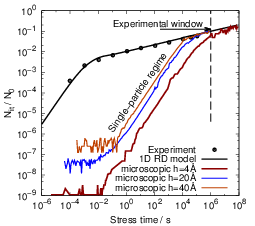A shift of the onset of the experimentally observed regime to earlier times at a realistic interstitial size requires a dramatic increase of either the hydrogen diffusion coefficient or the availability of free hydrogen near the interface. An increase of the hydrogen diffusion coefficient, however, breaks the dominance of H2 flux over the flux of atomic hydrogen and changes the predicted degradation away from the experimentally observed t16 towards t14. Increasing the availability of hydrogen at the interface by adjusting the ratio kf∕kr causes similar problems, as the H2 diffusion coefficient has to be lowered in order to give the same overall degradation.

This indicates that in the given microscopic model it is impossible to obtain the experimentally observed t16 degradation within the experimental window at a reasonable interstitial size.

#### 3.2.5Increased Interface Diffusion

The behavior predicted by the microscopic model is completely incompatible with any experimental data, while the description is much closer to the physical reality than the macroscopic RD model. Only two interpretations are possible to resolve this dilemma. Either the ability of the macroscopic RD model to fit degradation measurements has to be regarded as a mathematical artifact without physical meaning, or the structure of the Si/SiO2 interface somehow accelerates the lateral equilibration considerably. We investigated the second option more closely by considering first-principles calculations that have shown a lowering of diffusion barriers for hydrogen (molecules) along the SiSiO2-interface as compared to the bulk SiO2  . These findings indicate that the motion of hydrogen might proceed at a much higher rate at the interface. As a higher diffusivity at the interface aids the lateral equilibration, it might be the sought process that makes the one-dimensional RD model physically meaningful. To account for it in our microscopic model, we applied different diffusion coefficients DI and DB in the interface region and in the bulk, respectively.

 Figure 3.15: For increasing DI, the departure of hydrogen atoms from their dangling bond sites starts earlier, leading to an increased degradation at earlier times. Comparison to the classical RD model without H2 formation shows that competition for dangling bonds sets in after about 100s, leading to a t1∕4 degradation. The formation of H2 is slowly accelerated by the increased DI and only for DI →∞, the macroscopic behavior is obtained.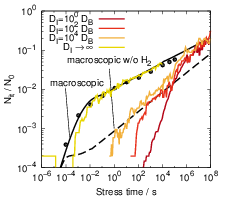As can be seen in Fig. 3.15, the increase of the interface diffusion coefficient accelerates the degradation during the initial phase as it increases the transport of hydrogen atoms away from their dangling bonds. Interestingly, even if the interface diffusion coefficient is increased by four orders of magnitude there is no t16 behavior visible, but instead the degradation takes on the typical t14 behavior of a hydrogen-only reaction-diffusion model. While the competition for dangling bonds sets in earlier for increased interface diffusion coefficients, the formation of H2 is not accelerated in the same way. Inspection of the atomic diffusion shows that the acceleration of the dimerization is much less pronounced as the liberated hydrogen atoms constantly leave the interface region into the bulk where the diffusion proceeds slower and the collision rate is reduced. Only in the limit of DI →∞ will the microscopic RD model match the experimentally observed behavior. Although these extremely high interface diffusion coefficients lack any physical justification, this is still closer to the physical reality than the assumption of immediate equilibration along the Si-SiO2-interface at any depth that is inherent to the usually employed one-dimensional macroscopic RD model.

As a side note we remark that in a real wafer, a nearly infinite diffusion coefficient along the SiSiO2-interface would make the hydrogen spread out through the waver during stress. This would again alter the degradation slope and give rise to cross-talk between neighboring devices that would be measurable, but has never been reported.

### 3.3Related Work

Three other scientific groups have put forward microscopic RD models recently  and interestingly those investigations find a reasonable agreement between their microscopic description and the macroscopic RD model. In the work of Islam et al.   the atomic description is basically equivalent to the work presented here but is built upon a one-dimensional foundation which carries the same implicit assumptions as the macroscopic model. Clearly this model cannot capture the effects discussed in this chapter as those are solely due to higher-dimensional effects. From a physical point of view, however, the one-dimensional approximation lacks justification considering the results presented above.

The work of Choi et al. considers the three-dimensional diffusion of the particles based on a grid-less stochastic formulation  . Although the degradation in that work seems to match the macroscopic RD model quite well at first sight, also strong discrepancies arise between the two for longer stress times. Interestingly, for situations where the approach presented above predicts a degradation far below the prediction of the macroscopic model, the degradation predicted by Choi et al. overshoots the macroscopic model considerably. Only for an enormous density of dangling bonds or a very large reaction radius the macroscopic behavior is obtained, in accord with our results. The degradation behavior in   initially follows Nit(t) = kft, which suggests that the depassivated hydrogen atoms instantly leave the reaction radius of their respective dangling bond. The following excessively high power-law exponent suggests that the repassivation of the silicon dangling bonds is somehow inhibited in this formulation. The most likely explanation for this behavior is a too low resolution of the time-stepping, in combination with the physically unjustifiable description of the diffusive motion.

The work of Panagopoulos et al. uses a grid-based stochastic RD model that seems to be compatible with our description. The surprisingly good agreement between their results and the macroscopic RD model may be an artifact of the employed method which is based on an adaptive time-stepping . Also, the paper states that the passivation reaction occurs if a hydrogen atom is “close” to a dangling bond. This indicates an artificial capture radius, but this is not explicitly stated. Also, the grid spacing is not given in the paper and its physical relevance is not discussed. However, as shown by our calculations, an unphysically large grid spacing strongly promotes bimolecular reactions and thus induces a degradation behavior that is (falsely) compatible with the macroscopic RD model.